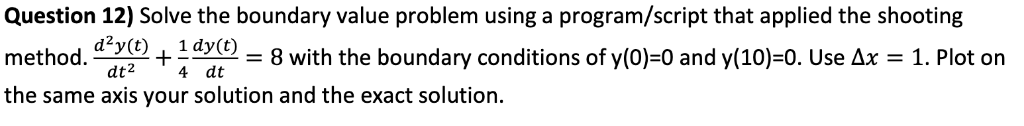# Question 12) Solve the boundary value problem using a program/script that applied the shooting me...

Please provide the program in Matlab.Question 12) Solve the boundary value problem using a program/script that applied the shooting method. (t) + y()-8 with the boundary conditions of y(0)-0 and y(10-0. Use ΔΧ-1. Plot on the same axis your solution and the exact solution dt2 t 4 4 dt

syms y(t)

ode = diff(y,x,2)+1/4 * diff(y,x)-8 == 0;

cond1 = y(0) ==0;

cond2 = y(10) ==0;

conds = [cond1 cond2];

ysol(t) = dsolv(ode,conds);

ysol = simplify(ysol)

##### Add Answer of: Question 12) Solve the boundary value problem using a program/script that applied the shooting me...
More Homework Help Questions Additional questions in this topic.

• #### In this problem we explore using Fourier series to solve nonhomogeneous boundary value problems. ...

Need Online Homework Help?